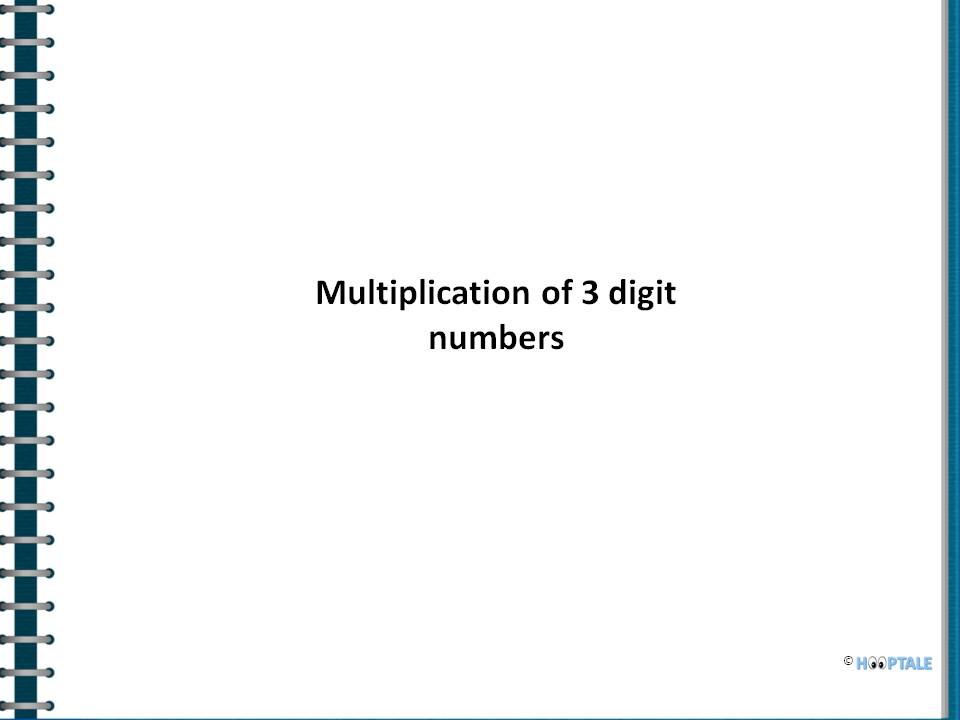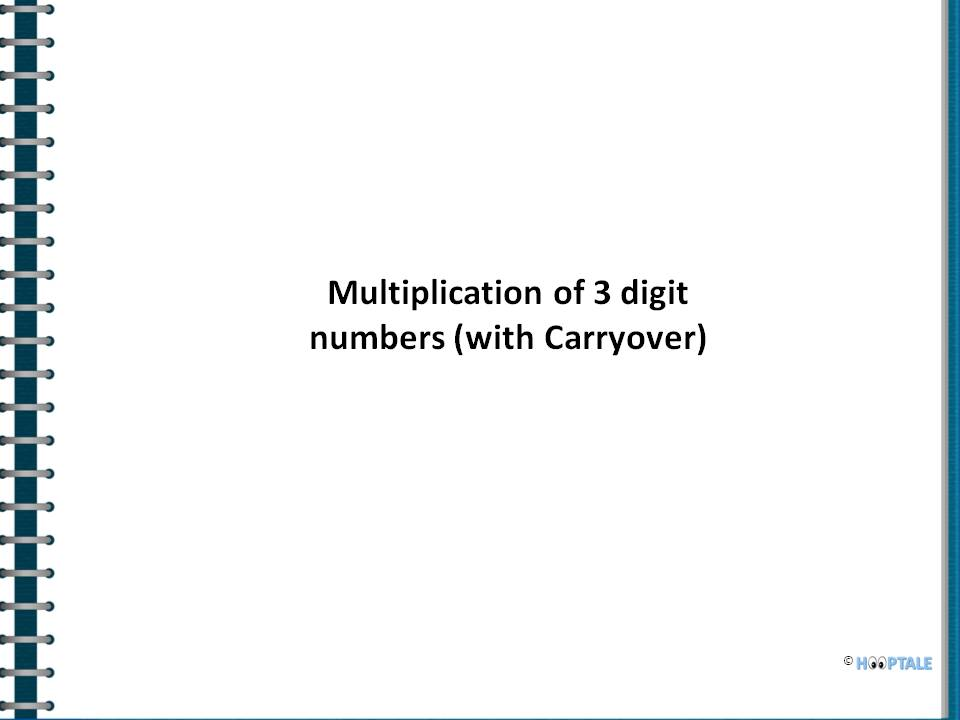# Multiplication Techniques based on Vedic Mathematics

##### 1. Multiplication of two 2 digit numbers

12*23

Follow these steps for numbers that do not return carryover,

 Step 1 Multiply the last digits of both the numbers  (2*3=6) Step 2 Cross multiply the digits of both numbers and add the cross multiplication (1*3+2*2=7) Step 3 Multiply the first digits of both the numbers (1*2=2)

Multiplication of 12 & 23 results to 276

### Multiplication of 2 digit numbers

space

57*23

Follow these steps in case of carryovers,

 Step 1 Multiply the last digits of both the numbers  (7*3=21) [Carryover 2 to step 2) Step 2 Cross multiply the digits of both numbers and add the cross multiplication (5*3+7*2=29)[Carryover 3 to step 3) Step 3 Multiply the first digits of both the numbers (5*2=10)

space

##### 2. Multiplication of any 3 digit numbers

103*213

Follow these steps for numbers that do not return carryover,

 Step 1 Multiply the last digits of both the numbers  (3*3=9) Step 2 Cross multiply the last 2 digits of both the numbers and add the cross multiplication (0*3+3*1=3) Step 3 Cross multiply first & last digits as well as multiply the middle digits of  both the numbers and add the results (1*3+0*1+3*2=9) Step 4 Cross multiply the first 2 digits of both the numbers and add the cross multiplication (1*1+0*2=2) Step 5 Multiply the first digits of both the numbers (1*2=2)

Multiplication of 103 & 213 results to 21939879*686

Follow these steps in case of carryovers,

 Step 1 Multiply the last digits of both the numbers  (9*6=54) [Carryover 5 to Step 2] Step 2 Cross multiply the last 2 digits of both the numbers and add the cross multiplication (7*6+9*8=114). [Carryover 11 to Step 3] Step 3 Cross multiply first & last digits as well as multiply the middle digits of  both the numbers and add the results (8*6+7*8+9*6=158) [Carryover 16 to Step 4] Step 4 Cross multiply the first 2 digits of both the numbers and add the cross multiplication (8*8+6*6=106). [Carryover 12 to Step 5] Step 5 Multiply the first digits of both the numbers (8*6=48)

Multiplication of 879 & 686 results to 602994space

##### 3. Multiplication of any two digit number with 11

25*11

Follow these steps for numbers that do not return carryover,

 Step 1 Separate the numbers as 2_5 Step 2 Add both digits & place the sum in the middle i.e. 2+5=7

75*11

Follow these steps in case of carryovers,

 Step 1 Separate the numbers as 7_5 Step 2 Add 7+5=12. Add carryover to the first digit i.e. 7+1=8

### Multi 2 digit with 11

space

##### 4. Multiplication of any number with 11

672827*11

 Step 1 Add 0 to the extreme right digit. Then add each digit to the respective preceding digit as below, 7+0=7 7+2=9 2+8=0 (1 will get carry over) 8+2+1=1 (1 will get carry over) 2+7+1=0 (the 1 will get carry over) 7+6+1=4 (the 1 will get carry over) 6+1=7

Multiplication of 672827 & 11 results to 7401097.